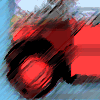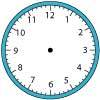Search by Topic

Resources tagged with Visualising similar to Reversals:

Filter by: Content type:
Age range:
Challenge level:

There are 176 results

Broad Topics > Using, Applying and Reasoning about Mathematics > VisualisingCuboids

Age 11 to 14 Challenge Level:

Find a cuboid (with edges of integer values) that has a surface area of exactly 100 square units. Is there more than one? Can you find them all?Frogs

Age 11 to 14 Challenge Level:

How many moves does it take to swap over some red and blue frogs? Do you have a method?Age 11 to 14 Challenge Level:

How many different symmetrical shapes can you make by shading triangles or squares?Tourism

Age 11 to 14 Challenge Level:

If you can copy a network without lifting your pen off the paper and without drawing any line twice, then it is traversable. Decide which of these diagrams are traversable.Reflecting Squarely

Age 11 to 14 Challenge Level:

In how many ways can you fit all three pieces together to make shapes with line symmetry?Triangles to Tetrahedra

Age 11 to 14 Challenge Level:

Imagine you have an unlimited number of four types of triangle. How many different tetrahedra can you make?Cubes Within Cubes Revisited

Age 11 to 14 Challenge Level:

Imagine starting with one yellow cube and covering it all over with a single layer of red cubes, and then covering that cube with a layer of blue cubes. How many red and blue cubes would you need?On the Edge

Age 11 to 14 Challenge Level:

If you move the tiles around, can you make squares with different coloured edges?Chess

Age 11 to 14 Challenge Level:

What would be the smallest number of moves needed to move a Knight from a chess set from one corner to the opposite corner of a 99 by 99 square board?Squares in Rectangles

Age 11 to 14 Challenge Level:

A 2 by 3 rectangle contains 8 squares and a 3 by 4 rectangle contains 20 squares. What size rectangle(s) contain(s) exactly 100 squares? Can you find them all?Hidden Rectangles

Age 11 to 14 Challenge Level:

Rectangles are considered different if they vary in size or have different locations. How many different rectangles can be drawn on a chessboard?Christmas Chocolates

Age 11 to 14 Challenge Level:

How could Penny, Tom and Matthew work out how many chocolates there are in different sized boxes?Isosceles Triangles

Age 11 to 14 Challenge Level:

Draw some isosceles triangles with an area of $9$cm$^2$ and a vertex at (20,20). If all the vertices must have whole number coordinates, how many is it possible to draw?Route to Infinity

Age 11 to 14 Challenge Level:

Can you describe this route to infinity? Where will the arrows take you next?Picturing Square Numbers

Age 11 to 14 Challenge Level:

Square numbers can be represented as the sum of consecutive odd numbers. What is the sum of 1 + 3 + ..... + 149 + 151 + 153?Picturing Triangular Numbers

Age 11 to 14 Challenge Level:

Triangular numbers can be represented by a triangular array of squares. What do you notice about the sum of identical triangle numbers?Take Ten

Age 11 to 14 Challenge Level:

Is it possible to remove ten unit cubes from a 3 by 3 by 3 cube so that the surface area of the remaining solid is the same as the surface area of the original?Clocked

Age 11 to 14 Challenge Level:

Is it possible to rearrange the numbers 1,2......12 around a clock face in such a way that every two numbers in adjacent positions differ by any of 3, 4 or 5 hours?Rati-o

Age 11 to 14 Challenge Level:

Points P, Q, R and S each divide the sides AB, BC, CD and DA respectively in the ratio of 2 : 1. Join the points. What is the area of the parallelogram PQRS in relation to the original rectangle?Pattern Power

Age 5 to 14

Mathematics is the study of patterns. Studying pattern is an opportunity to observe, hypothesise, experiment, discover and create.Dotty Triangles

Age 11 to 14 Challenge Level:

Imagine an infinitely large sheet of square dotty paper on which you can draw triangles of any size you wish (providing each vertex is on a dot). What areas is it/is it not possible to draw?Framed

Age 11 to 14 Challenge Level:

Seven small rectangular pictures have one inch wide frames. The frames are removed and the pictures are fitted together like a jigsaw to make a rectangle of length 12 inches. Find the dimensions of. . . .Convex Polygons

Age 11 to 14 Challenge Level:

Show that among the interior angles of a convex polygon there cannot be more than three acute angles.Coloured Edges

Age 11 to 14 Challenge Level:

The whole set of tiles is used to make a square. This has a green and blue border. There are no green or blue tiles anywhere in the square except on this border. How many tiles are there in the set?Zooming in on the Squares

Age 7 to 14

Start with a large square, join the midpoints of its sides, you'll see four right angled triangles. Remove these triangles, a second square is left. Repeat the operation. What happens?Sea Defences

Age 7 to 14 Challenge Level:

These are pictures of the sea defences at New Brighton. Can you work out what a basic shape might be in both images of the sea wall and work out a way they might fit together?All in the Mind

Age 11 to 14 Challenge Level:

Imagine you are suspending a cube from one vertex and allowing it to hang freely. What shape does the surface of the water make around the cube?Jam

Age 14 to 16 Challenge Level:

To avoid losing think of another very well known game where the patterns of play are similar.How Many Dice?

Age 11 to 14 Challenge Level:

A standard die has the numbers 1, 2 and 3 are opposite 6, 5 and 4 respectively so that opposite faces add to 7? If you make standard dice by writing 1, 2, 3, 4, 5, 6 on blank cubes you will find. . . .Tetra Square

Age 11 to 14 Challenge Level:

ABCD is a regular tetrahedron and the points P, Q, R and S are the midpoints of the edges AB, BD, CD and CA. Prove that PQRS is a square.Konigsberg Plus

Age 11 to 14 Challenge Level:

Euler discussed whether or not it was possible to stroll around Koenigsberg crossing each of its seven bridges exactly once. Experiment with different numbers of islands and bridges.Age 11 to 14 Challenge Level:

Four rods, two of length a and two of length b, are linked to form a kite. The linkage is moveable so that the angles change. What is the maximum area of the kite?Age 11 to 14 Challenge Level:

Can you mark 4 points on a flat surface so that there are only two different distances between them?Constructing Triangles

Age 11 to 14 Challenge Level:

Generate three random numbers to determine the side lengths of a triangle. What triangles can you draw?Seven Squares

Age 11 to 14 Challenge Level:

Watch these videos to see how Phoebe, Alice and Luke chose to draw 7 squares. How would they draw 100?3D Stacks

Age 7 to 14 Challenge Level:

Can you find a way of representing these arrangements of balls?Auditorium Steps

Age 7 to 14 Challenge Level:

What is the shape of wrapping paper that you would need to completely wrap this model?Troublesome Dice

Age 11 to 14 Challenge Level:

When dice land edge-up, we usually roll again. But what if we didn't...?Eight Hidden Squares

Age 7 to 14 Challenge Level:

On the graph there are 28 marked points. These points all mark the vertices (corners) of eight hidden squares. Can you find the eight hidden squares?There and Back Again

Age 11 to 14 Challenge Level:

Bilbo goes on an adventure, before arriving back home. Using the information given about his journey, can you work out where Bilbo lives?Square Coordinates

Age 11 to 14 Challenge Level:

A tilted square is a square with no horizontal sides. Can you devise a general instruction for the construction of a square when you are given just one of its sides?An Unusual Shape

Age 11 to 14 Challenge Level:

Can you maximise the area available to a grazing goat?Buses

Age 11 to 14 Challenge Level:

A bus route has a total duration of 40 minutes. Every 10 minutes, two buses set out, one from each end. How many buses will one bus meet on its way from one end to the other end?Bands and Bridges: Bringing Topology Back

Age 7 to 14

Lyndon Baker describes how the Mobius strip and Euler's law can introduce pupils to the idea of topology.Coordinate Patterns

Age 11 to 14 Challenge Level:

Charlie and Alison have been drawing patterns on coordinate grids. Can you picture where the patterns lead?Sliding Puzzle

Age 11 to 16 Challenge Level:

The aim of the game is to slide the green square from the top right hand corner to the bottom left hand corner in the least number of moves.Khun Phaen Escapes to Freedom

Age 11 to 14 Challenge Level:

Slide the pieces to move Khun Phaen past all the guards into the position on the right from which he can escape to freedom.Dice, Routes and Pathways

Age 5 to 14

This article for teachers discusses examples of problems in which there is no obvious method but in which children can be encouraged to think deeply about the context and extend their ability to. . . .The Old Goats

Age 11 to 14 Challenge Level:

A rectangular field has two posts with a ring on top of each post. There are two quarrelsome goats and plenty of ropes which you can tie to their collars. How can you secure them so they can't. . . .Right Time

Age 11 to 14 Challenge Level:

At the time of writing the hour and minute hands of my clock are at right angles. How long will it be before they are at right angles again?